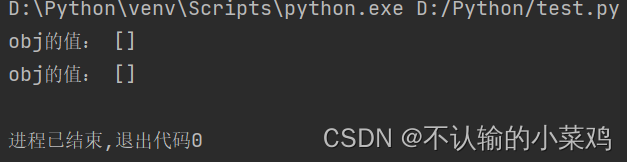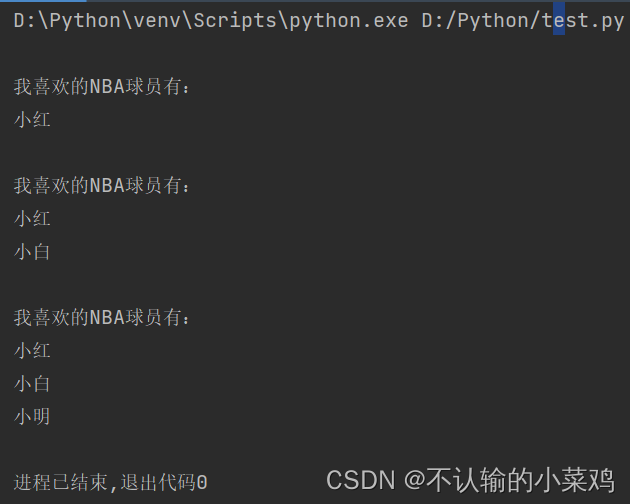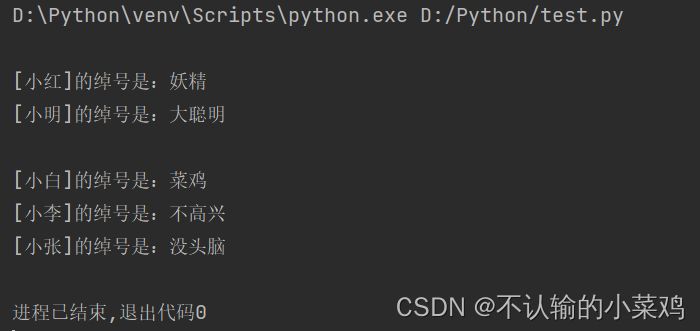﻿ Python中的函数参数传递问题_python_脚本之家
python# Python中的函数参数传递问题

## 一、前言## 二、了解形式参数和实际参数

### 1.通过作用理解

• 形式传参：在定义函数时，函数名后面括号中的参数为“形式参数”，也称形参。
• 实际参数：在调用一个函数时，函数名后面括号中的参数为“实际参数”。也就是将函数的调用者提供给函数的参数称为实际参数，也称为实参。• 当实参为不可变对象时，进行的是值传递
• 当实参为可变对象时，进行的是引用传递

• 进行值传递后，改变形参的值，实参的值不变
• 进行引用传递后，改变形参的值，实参的值也一同改变

```# 定义函数
def demo(obj):
print("原值:", obj)
obj += obj

# 调用函数
print("==========值传递==========")
mot = "唯有在被追赶的时候，你才能真正地奔跑"
print("函数调用前:", mot)
demo(mot)  # 采用不可变对象-字符串
print("函数调用后:", mot)

print("==========引用传递==========")
list1 = ["小白", "小名", "小红"]
print("函数调用前:", list1)
demo(list1)  # 采用可变对象-列表
print("函数调用后:", list1)
```## 三、位置参数

### 1.数量必须与定义时一致

```def fun_bmi(person, height, weight):
"""
功能：根据身高和体重计算BMI指数
:param person: 姓名
:param height: 身高，单位：米
:param weight: 体重，单位：千克
"""
print(person + "的身高：" + str(height) + "米\t 体重：" + str(weight) + "千克")
bmi = weight / (height * height)  # 用于计算BMI指数，公式为“体重/身高的平方”
print(person + "的BMI指数为：" + str(bmi))

# 判断身材是否合理
if bmi < 18.5:
print("您的体重过轻")
if 18.5 <= bmi < 24.9:
print("正常范围，注意保持")
if 24.9 <= bmi < 29.9:
print("您的体重过重")
if bmi > 29.9:
print("肥胖")

fun_bmi("路人甲", 183)
```### 2.位置必须与定义时一致

• 抛出TypeError异常产
• 生结果与预期不符的问题

```def fun_bmi(person, height, weight):
"""
功能：根据身高和体重计算BMI指数
:param person: 姓名
:param height: 身高，单位：米
:param weight: 体重，单位：千克
"""
print(person + "的身高：" + str(height) + "米\t 体重：" + str(weight) + "千克")
bmi = weight / (height * height)  # 用于计算BMI指数，公式为“体重/身高的平方”
print(person + "的BMI指数为：" + str(bmi))

# 判断身材是否合理
if bmi < 18.5:
print("您的体重过轻")
if 18.5 <= bmi < 24.9:
print("正常范围，注意保持")
if 24.9 <= bmi < 29.9:
print("您的体重过重")
if bmi > 29.9:
print("肥胖")

fun_bmi("路人甲", 60, 183)
```## 四、关键字参数

```fun_bmi(height=1.83, weight=60, person="路人甲")
```## 五、为参数设置默认值

`def functionname(...,[parameter1 = defaultvalue1]):[functionbody]`

• `functionname`：函数名称，在调用时使用
• `parameter1 = defaultvalue1`：可选参数，用于指定向函数中传递的出纳室，并且为该参数设置默认值为defaultvalue1
• `functionbody`：可选参数，用于指定函数体，即该函数被调用后，要执行的功能代码

```def demo(obj=[]):
print("obj的值：", obj)
obj.append(1)
```

`demo() # 调用函数````demo() # 调用函数
demo() # 调用函数``````def demo(obj=None):  # 定义一个函数
if obj == None:  # 判断是否为空
obj = []
print("obj的值：", obj)  # 输出obj值
obj.append(1)  # 连续调用并输出
```## 六、可变参数

• *`parameter`
• **`parameter`

### 1.*parameter

```def printplayer(*name):  # 定义输出我喜欢的NBA球员的函数
print("\n我喜欢的NBA球员有：")
for item in name:
print(item)  # 输出球员名称
```

```printplayer("小红")
printplayer("小红", "小白")
printplayer("小红", "小白", "小明")
```### 2.**parameter

```def printsign(**sign):  # 定义输出姓名和绰号的函数
print()  # 输出一个空行
for key, value in sign.items():  # 遍历字典
print("[" + key + "]的绰号是：" + value)  # 输出组合获得信息
```

```printsign(小红="妖精", 小明="大聪明")
printsign(小白="菜鸡", 小李="不高兴", 小张="没头脑")
``````dict1 = {"小白": "菜鸡", "小李": "不高兴", "小张": "没头脑"}  # 定义一个字典
printsign(**dict1)  # 通过字典指定函数的可变参
```